# 开什么玩笑？股票价格如何经得起AI的推敲？| 技术头条【导语】用深度学习预测股票价格不是一个新话题，随着技术的不断发展，大家一直在不断尝试新技术。这次教程中，作者设计了一个强强联合型模型来预测股票价格，为什么这么形容？作者设计了一个 GAN 模型，其生成网络为 LSTM 模型用来预测时间序列数据、CNN 模型作判别网络，用 BERT 模型作为情绪分析模型。带有高斯过程的贝叶斯优化和深度强化学习方法来获得 GAN 的超参数。为什么创建这样的组合？AI科技大本营都将在下面的内容中为大家进行一一解答。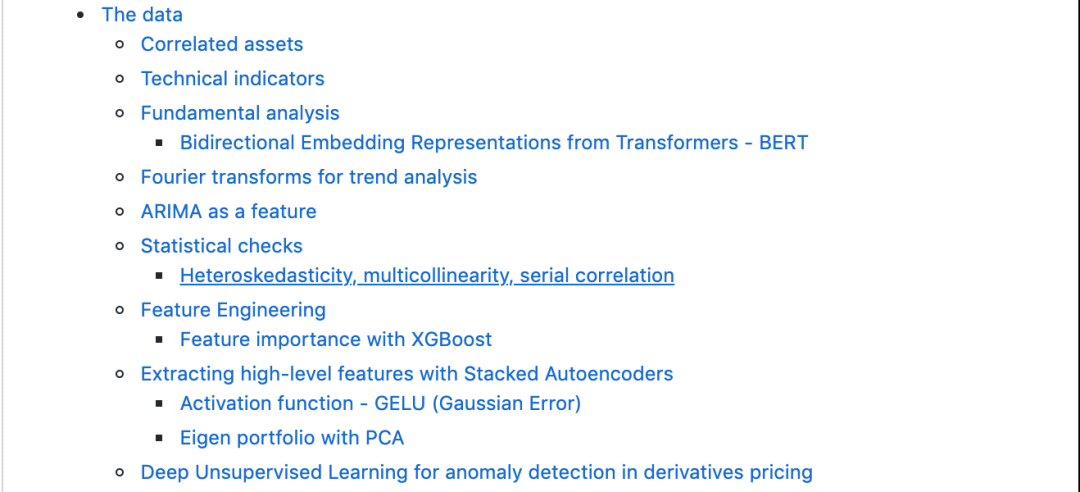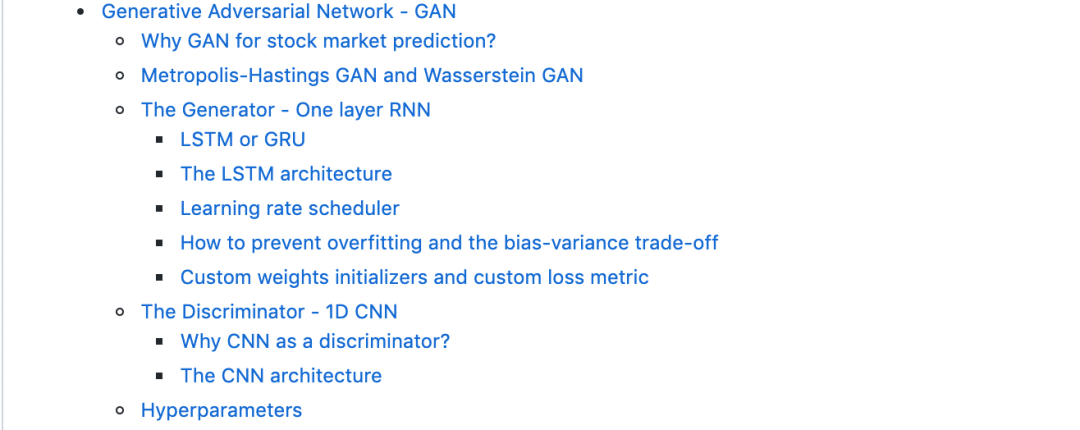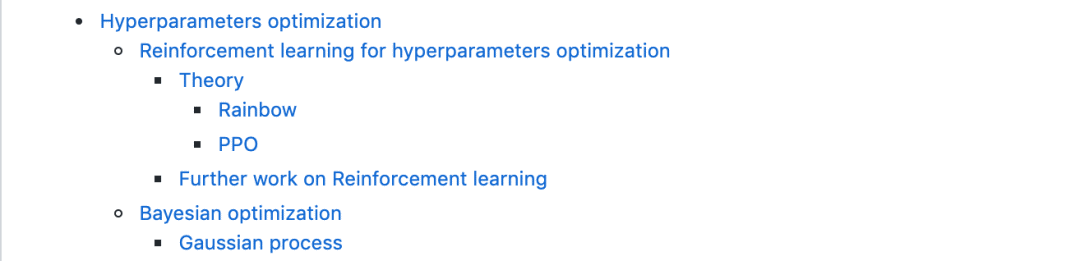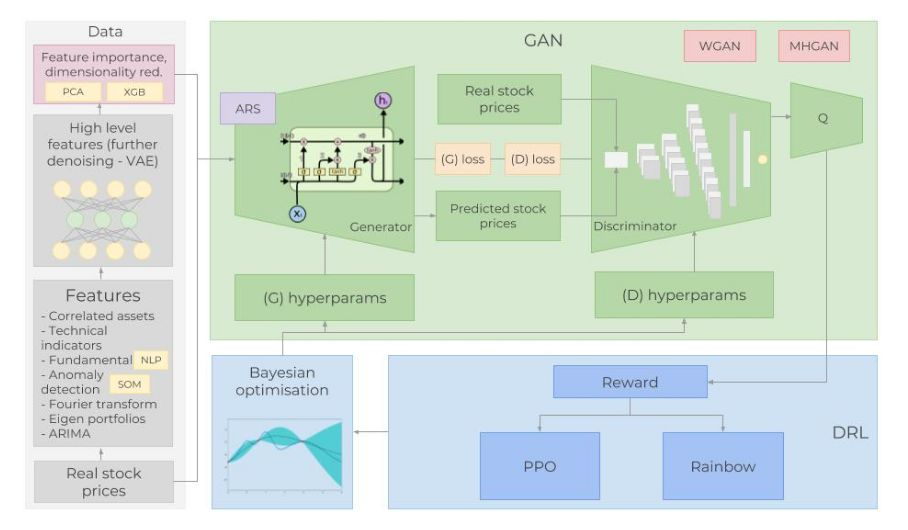图：完整体系结构概览

a.相关资产：涉及商品、外汇、指数、固定收益证券等各类资产数据；影响高盛公司股票价格趋势的外部因素又有很多，并且很复杂，包括竞争对手、客户、全球经济、地缘政治形势、财政和货币政策等等，这些因素还会相互产生影响。选择合适的相关资产是非常重要的：

（1）首先是和 GS 相似的公司，如将摩根大通（JPMorgan Chase）和摩根士丹利（Morgan Stanley）等加入数据集。

（2）作为一家投资银行，高盛依赖于全球经济，需要关注全球经济指数和 libor 利率。

（3）每日波动指数(VIX)。

（4）综合指数，如 NASDAQ 和 NYSE（美国）、FTSE 100（英国）、日经指数 225（日本）、恒生指数和 BSE Sensex（APAC）指数。

（5）货币，全球贸易多次反映在货币流动中，使用一篮子货币（如美元-日元、英镑-美元等）作为特征。

b.技术指标：许多投资人都会关注技术指标，在这里，把最受欢迎的指标作为独立特征，包括 7 天和 21 天波动平均值、指数波动平均、Momentum、MACD 等 12项技术指标。

def get_technical_indicators(dataset):    # Create 7 and 21 days Moving Average    dataset['ma7'] = dataset['price'].rolling(window=7).mean()    dataset['ma21'] = dataset['price'].rolling(window=21).mean()        # Create MACD    dataset['26ema'] = pd.ewma(dataset['price'], span=26)    dataset['12ema'] = pd.ewma(dataset['price'], span=12)    dataset['MACD'] = (dataset['12ema']-dataset['26ema'])    # Create Bollinger Bands    dataset['20sd'] = pd.stats.moments.rolling_std(dataset['price'],20)    dataset['upper_band'] = dataset['ma21'] + (dataset['20sd']*2)    dataset['lower_band'] = dataset['ma21'] - (dataset['20sd']*2)        # Create Exponential moving average    dataset['ema'] = dataset['price'].ewm(com=0.5).mean()        # Create Momentum    dataset['momentum'] = dataset['price']-1        return datasetdataset_TI_df = get_technical_indicators(dataset_ex_df[['GS']])dataset_TI_df.head()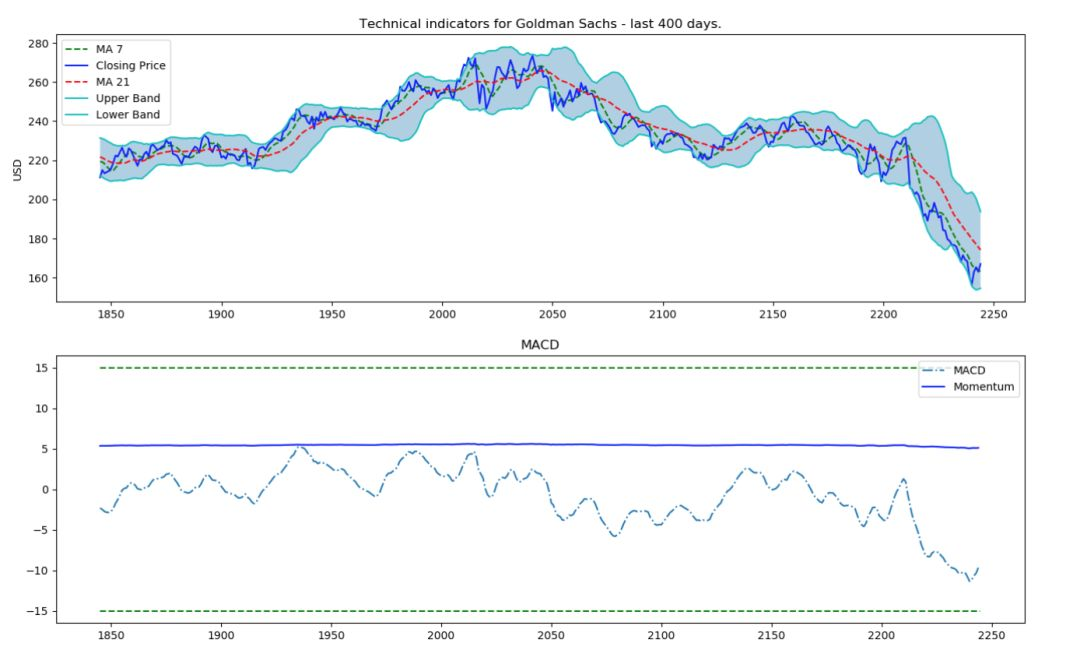c.基本面分析：无论股票涨跌，这都是一个非常重要的数据。分析时会用到两个特征：公司业绩报告和新闻将引导的一些趋势，因此通过分析新闻来准确预测市场的情绪也是一项非常重要的工作，所以这次的方法中，将使用 BERT 来构建情绪分析模型，提取股票新闻中的情绪倾向。最后采用 sigmoid 归一化，结果介于 0 到 1 之间，（0 表示负面情绪，1 表示正面情绪），每一天都会创建一个平均每日分数作为一个特征添加。

d.傅里叶变换：利用每日收盘价，创建傅立叶变换，以获得几个长期和短期趋势。使用这些变换消除大量的噪声，获得真实股票波动的近似值。有了趋势近似，可以帮助 LSTM 网络更准确地选择其预测趋势。

data_FT = dataset_ex_df[['Date', 'GS']]close_fft = np.fft.fft(np.asarray(data_FT['GS'].tolist()))fft_df = pd.DataFrame({'fft':close_fft})fft_df['absolute'] = fft_df['fft'].apply(lambda x: np.abs(x))fft_df['angle'] = fft_df['fft'].apply(lambda x: np.angle(x))plt.figure(figsize=(14, 7), dpi=100)fft_list = np.asarray(fft_df['fft'].tolist())for num_ in [3, 6, 9, 100]:    fft_list_m10= np.copy(fft_list); fft_list_m10[num_:-num_]=0    plt.plot(np.fft.ifft(fft_list_m10), label='Fourier transform with {} components'.format(num_))plt.plot(data_FT['GS'],  label='Real')plt.xlabel('Days')plt.ylabel('USD')plt.title('Figure 3: Goldman Sachs (close) stock prices & Fourier transforms')plt.legend()plt.show()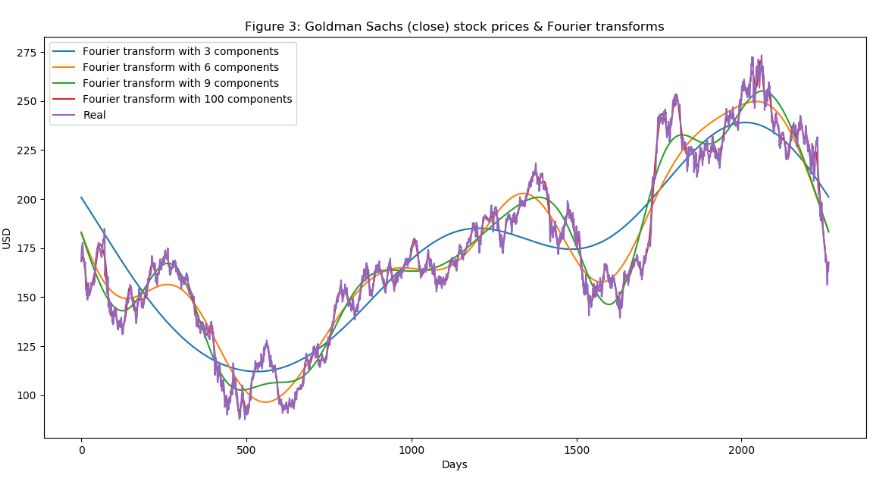图：高盛股票的傅里叶变换

e.ARIMA：这是预测时间序列数据未来值的最流行技术之一。

from pandas import read_csvfrom pandas import datetimefrom statsmodels.tsa.arima_model import ARIMAfrom sklearn.metrics import mean_squared_errorX = series.valuessize = int(len(X) * 0.66)train, test = X[0:size], X[size:len(X)]history = [x for x in train]predictions = list()for t in range(len(test)):    model = ARIMA(history, order=(5,1,0))    model_fit = model.fit(disp=0)    output = model_fit.forecast()    yhat = output    predictions.append(yhat)    obs = test[t]    history.append(obs)error = mean_squared_error(test, predictions)print('Test MSE: %.3f' % error)Test MSE: 10.151# Plot the predicted (from ARIMA) and real pricesplt.figure(figsize=(12, 6), dpi=100)plt.plot(test, label='Real')plt.plot(predictions, color='red', label='Predicted')plt.xlabel('Days')plt.ylabel('USD')plt.title('Figure 5: ARIMA model on GS stock')plt.legend()plt.show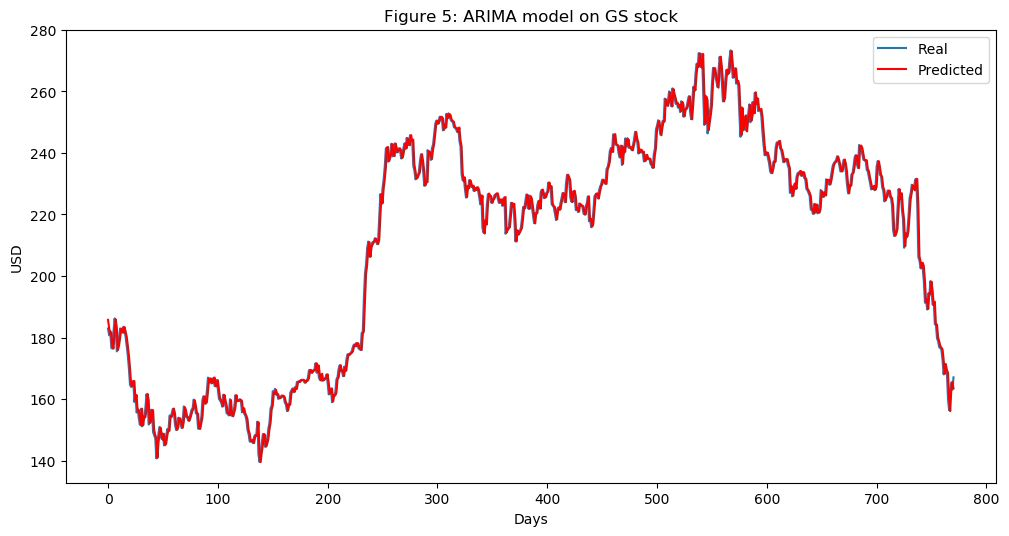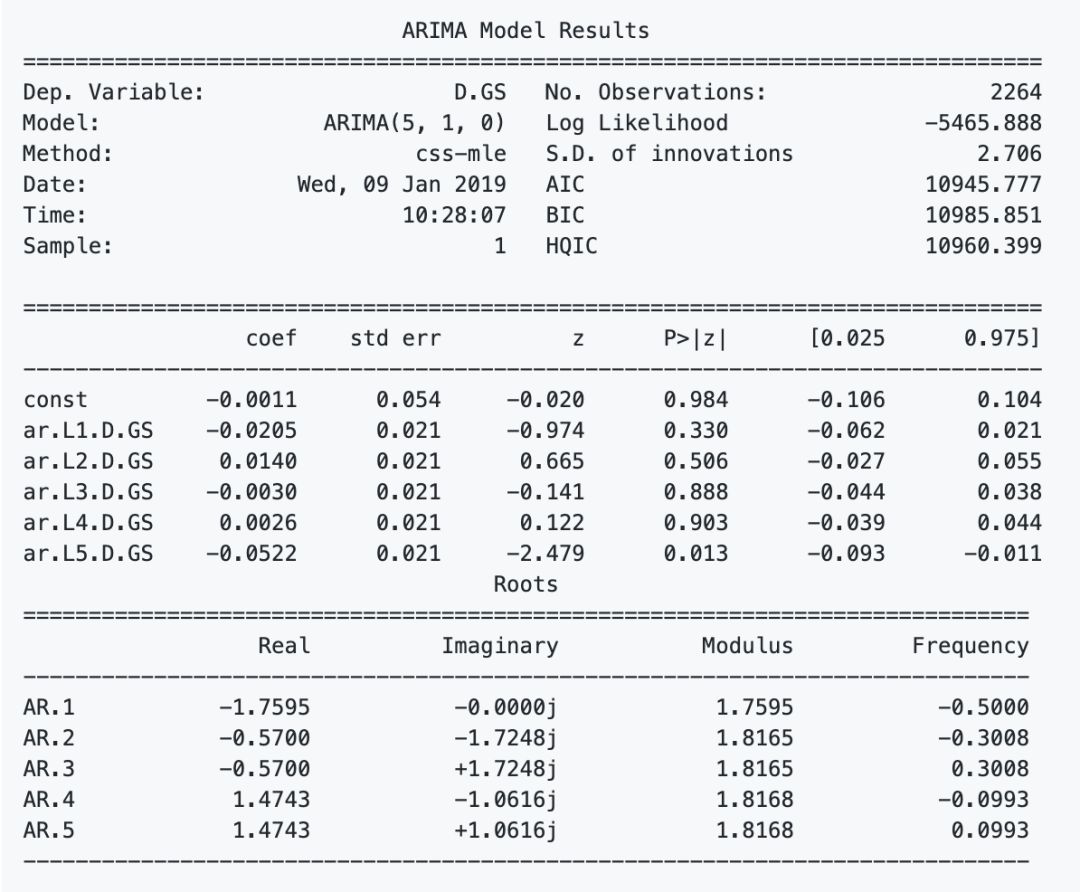f.Stacked autoencoders （栈式自动编码器）：上面提到的一些特征是研究人员经过几十年的研究发现的，但是还是会忽视一些隐藏的关联特征，由此，Stacked autoencoders  就可以解决这个问题，通过学习每个隐藏层，发现更多新特征（可能有些是我们无法发现，理解的）。这次没有把 RELU 作为激活函数，而是使用了 GELU，也可以用于 BERT 模型中。至于为什么选择 GELU，大家可以在原文中看到作者给出的和 RELU 对比的实例。

g.深度无监督学习：用于期权定价中的异常检测，将再使用一个特征：每天都会增加高盛股票90天看涨期权的价格。期权定价本身结合了很多数据。期权合约的价格取决于股票的未来价值(分析师也试图预测价格，以便为看涨期权得出最准确的价格)。使用深度无监督学习(自组织映射)，尝试发现出现异常的每日价格。异常(如价格的剧烈变化)可能表明出现了一个事件，这有助于LSTM了解整体股票模式。

from utils import *import timeimport numpy as npfrom mxnet import nd, autograd, gluonfrom mxnet.gluon import nn, rnnimport mxnet as mximport datetimeimport seaborn as snsimport matplotlib.pyplot as plt%matplotlib inlinefrom sklearn.decomposition import PCAimport mathfrom sklearn.preprocessing import MinMaxScalerfrom sklearn.metrics import mean_squared_errorfrom sklearn.preprocessing import StandardScalerimport xgboost as xgbfrom sklearn.metrics import accuracy_scoreimport warningswarnings.filterwarnings("ignore")context = mx.cpu(); model_ctx=mx.cpu()mx.random.seed(1719)Note: The purpose of this section (3. The Data) is to show the data preprocessing and to give rationale for using different sources of data, hence I will only use a subset of the full data (that is used for training).def parser(x):    return datetime.datetime.strptime(x,'%Y-%m-%d')dataset_ex_df = pd.read_csv('data/panel_data_close.csv', header=0, parse_dates=, date_parser=parser)dataset_ex_df[['Date', 'GS']].

h.对数据的“质量”进行统计检查：确保数据质量对模型来说非常重要，因此要执行以下几个简单的检验，如异方差、多重共线性、Serial correlation 等。

i.确定特征重要性：采用 XGBoost 算法。这么多的特征，必须考虑是否所有这些都真正地指示了 GS 股票波动方向。例如，数据集中包括其变化可能意味着经济变化的 LIBOR，而这又可能暗示 GS 股票将会发生波动，因此需要对此预测进行测试，在众多的测试方法中，本教程中选择了 XGBoost，其在分类和回归问题上都提供了很好的结果。

def get_feature_importance_data(data_income):    data = data_income.copy()    y = data['price']    X = data.iloc[:, 1:]        train_samples = int(X.shape * 0.65)     X_train = X.iloc[:train_samples]    X_test = X.iloc[train_samples:]    y_train = y.iloc[:train_samples]    y_test = y.iloc[train_samples:]        return (X_train, y_train), (X_test, y_test)# Get training and test data(X_train_FI, y_train_FI), (X_test_FI, y_test_FI) = get_feature_importance_data(dataset_TI_df)regressor = xgb.XGBRegressor(gamma=0.0,n_estimators=150,base_score=0.7,colsample_bytree=1,learning_rate=0.05)xgbModel = regressor.fit(X_train_FI,y_train_FI, \                         eval_set = [(X_train_FI, y_train_FI), (X_test_FI, y_test_FI)], \                         verbose=False)eval_result = regressor.evals_result()training_rounds = range(len(eval_result['validation_0']['rmse']))

plt.figure(figsize=(15, 5))plt.subplots_adjust(left=None, bottom=None, right=None, top=None, wspace=.5, hspace=None)ranges_ = (-10, 3, .25)plt.subplot(1, 2, 1)plt.plot([i for i in np.arange(*ranges_)], [relu(i) for i in np.arange(*ranges_)], label='ReLU', marker='.')plt.plot([i for i in np.arange(*ranges_)], [gelu(i) for i in np.arange(*ranges_)], label='GELU')plt.hlines(0, -10, 3, colors='gray', linestyles='--', label='0')plt.title('Figure 7: GELU as an activation function for autoencoders')plt.ylabel('f(x) for GELU and ReLU')plt.xlabel('x')plt.legend()plt.subplot(1, 2, 2)plt.plot([i for i in np.arange(*ranges_)], [lrelu(i) for i in np.arange(*ranges_)], label='Leaky ReLU')plt.hlines(0, -10, 3, colors='gray', linestyles='--', label='0')plt.ylabel('f(x) for Leaky ReLU')plt.xlabel('x')plt.title('Figure 8: LeakyReLU')plt.legend()plt.sho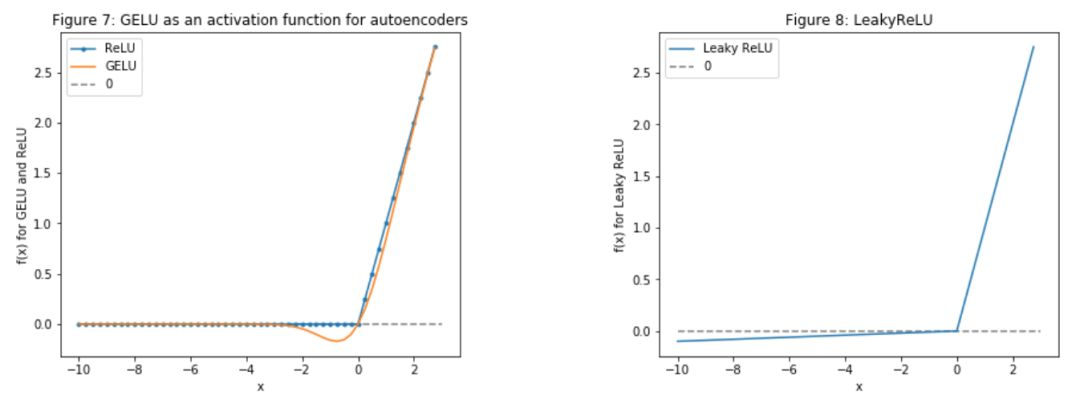plt.figure(figsize=(14, 5), dpi=100)plt.plot(dataset_ex_df['Date'], dataset_ex_df['GS'], label='Goldman Sachs stock')plt.vlines(datetime.date(2016,4, 20), 0, 270, linestyles='--', colors='gray', label='Train/Test data cut-off')plt.xlabel('Date')plt.ylabel('USD')plt.title('Figure 2: Goldman Sachs stock price')plt.legend()plt.show()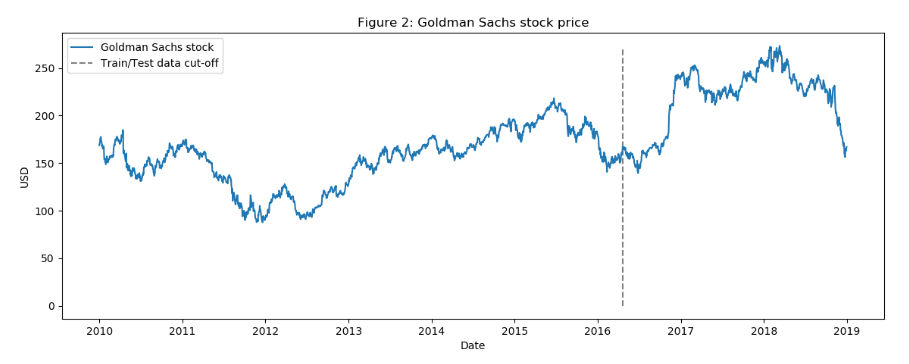图：过去9年高盛股价的波动

num_training_days = int(dataset_ex_df.shape*.7)print('Number of training days: {}. Number of test days: {}.'.format(num_training_days, \                                                                    dataset_ex_df.shape-num_training_days))Number of training days: 1585. Number of test days: 680.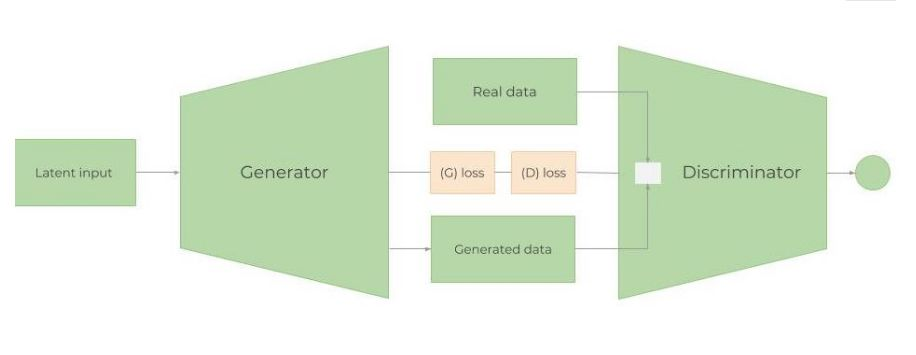图：GAN 架构

1、为什么采用GAN进行股市预测？

GAN 最多被应用在创作逼真的图像、画作和视频剪辑等。对预测时间序列数据的应用并不多。但这两者的思想都是类似的。我们希望预测未来的股票价格，GS 的股票波动和行为应该大致相同(除非开始以完全不同的方式运作，或者经济急剧变化)。因此，希望“生成”的数据与已经拥有的历史交易数据分布相似，当然不是完全相同。在这个例子中将使用 LSTM 作为时间序列生成模型，CNN 作为判别模型。

2、Metropolis-Hastings GAN 和 Wasserstein GAN

（1）Metropolis-Hastings GAN：与传统的 GAN 相比，Uber 团队最近提出一种新改进的 GAN 模型——Metropolis-Hastings GAN （MHGAN），它有点类似于谷歌和加州大学伯克利分校提出的Discriminator Rejection Sampling。通常情况下，在训练完GAN之后就不再使用 D 了。然而，MHGAN 和 DRS 试图使用 D 来选择由 G 生成的接近真实的样本。

（2）Wasserstein GAN：训练 GAN 是相当困难的。模型可能永远不会收敛，模式崩溃也很容易发生。，通过 Wasserstein GAN 尝试解决这个问题。KL 距离和 JS 距离是两种常用的分布，而 WGAN 使用的是 Wasserstein distanc。

3、生成模型：单层RNN

（1）LSTM 还是 GRU？

（2）LSTM 体系结构

LSTM架构非常简单：一个LSTM层，包含112个输入单元(数据集中有112个特征)和500个隐藏单元；一个以每日股价为输出的 Dense 层；采用 Xavier 初始化，使用 L1 损失函数

gan_num_features = dataset_total_df.shapesequence_length = 17class RNNModel(gluon.Block):    def __init__(self, num_embed, num_hidden, num_layers, bidirectional=False, \                 sequence_length=sequence_length, **kwargs):        super(RNNModel, self).__init__(**kwargs)        self.num_hidden = num_hidden        with self.name_scope():            self.rnn = rnn.LSTM(num_hidden, num_layers, input_size=num_embed, \                                bidirectional=bidirectional, layout='TNC')                        self.decoder = nn.Dense(1, in_units=num_hidden)        def forward(self, inputs, hidden):        output, hidden = self.rnn(inputs, hidden)        decoded = self.decoder(output.reshape((-1, self.num_hidden)))        return decoded, hidden        def begin_state(self, *args, **kwargs):        return self.rnn.begin_state(*args, **kwargs)    lstm_model = RNNModel(num_embed=gan_num_features, num_hidden=500, num_layers=1)lstm_model.collect_params().initialize(mx.init.Xavier(), ctx=mx.cpu())trainer = gluon.Trainer(lstm_model.collect_params(), 'adam', {'learning_rate': .01})loss = glu

（3）学习率调度器

class TriangularSchedule():    def __init__(self, min_lr, max_lr, cycle_length, inc_fraction=0.5):             self.min_lr = min_lr        self.max_lr = max_lr        self.cycle_length = cycle_length        self.inc_fraction = inc_fraction            def __call__(self, iteration):        if iteration <= self.cycle_length*self.inc_fraction:            unit_cycle = iteration * 1 / (self.cycle_length * self.inc_fraction)        elif iteration <= self.cycle_length:            unit_cycle = (self.cycle_length - iteration) * 1 / (self.cycle_length * (1 - self.inc_fraction))        else:            unit_cycle = 0        adjusted_cycle = (unit_cycle * (self.max_lr - self.min_lr)) + self.min_lr        return adjusted_cycleclass CyclicalSchedule():    def __init__(self, schedule_class, cycle_length, cycle_length_decay=1, cycle_magnitude_decay=1, **kwargs):        self.schedule_class = schedule_class        self.length = cycle_length        self.length_decay = cycle_length_decay        self.magnitude_decay = cycle_magnitude_decay        self.kwargs = kwargs        def __call__(self, iteration):        cycle_idx = 0        cycle_length = self.length        idx = self.length        while idx <= iteration:            cycle_length = math.ceil(cycle_length * self.length_decay)            cycle_idx += 1            idx += cycle_length        cycle_offset = iteration - idx + cycle_length                schedule = self.schedule_class(cycle_length=cycle_length,**self.kwargs)        return schedule(cycle_offset) *self.magnitude_decay**cycle_idx

（4）防止过拟合与偏差-方差权衡

a.确保数据质量

b.正则化，或权重惩罚：最常用的两种正则化技术是L1 和 L2 正则法。L1对离散值更有鲁棒性，当数据稀疏时使用，可得到特征重要性。因此，在股票价格预测这个应用案例中将使用 L1 正则法。

c.Dropout。Dropout层随机删除隐藏层中的节点。

d.Dense-sparse-dense training

e.提前停止.

（5）权衡偏差-方差

a.偏差（Bias）：偏差衡量一个经过训练的（训练数据集）算法对未见数据的概括能力。高偏差(欠拟合)意味着模型在隐藏数据上不能很好地工作。

b.方差（Variance）：方差衡量模型对数据集变化的敏感性。高方差意味着过拟合。

4、 一维 CNN 判别模型

（1）为何采用CNN作为判别模型？

CNN 网络在提取隐藏特征等工作上具有优势，那如何应用于这个任务中？大家不妨尝试一下，数据点行程小趋势，小趋势行程大趋势，趋势反之形成模式，而 CNN 在此用检测特征的能力来提取 GS 股价趋势中的模式信息。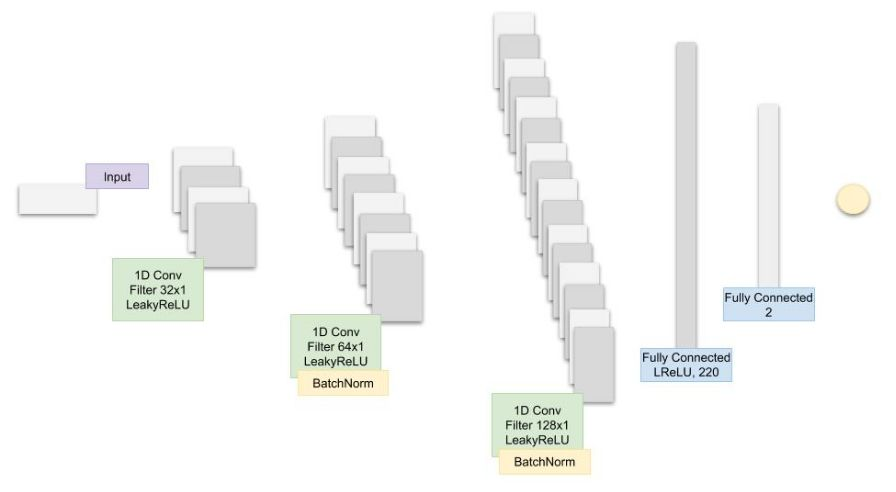图：本文提出的 CNN 模型的体系结构。

（1）跟踪和优化的超参数是：

batch_size：LSTM 和 CNN 的 Batch 大小

cnn_lr：CNN 的学习率

strides：CNN 的跨步卷积数

lrelu_alpha：GAN 中 LeakyReLU 的 Alpha 值

batchnorm_momentum：CNN Batch 正则化的 momentum

kernel_size：1 CNN 的内核大小

dropout：LSTM 中的 Dropout 层

filters：过滤器的初始数目

epoch = 200

（2）超参数优化

（3）超参数优化中的强化学习

（4）强化学习理论

a.Q-Learning：一种基于Q-Learning的非策略深度强化学习算法，它将7种算法结合在一起：DQN、Double Q Learning（双QL）、Prioritized replay、决斗网络(Dueling networks)、多步学习、分布式RL、噪声网络（Noisy Nets）。在Q-Learning中，学习价值从某一状态采取行动。Q 值采取行动后的预期回报。

b.策略优化：这里采用近端策略优化（Proximal Policy Optimization, PPO），在决策优化中，学习从某一状态采取的行动。(如果使用诸如Actor/Critic之类的方法，也会了解处于给定状态的价值。

（5）贝叶斯优化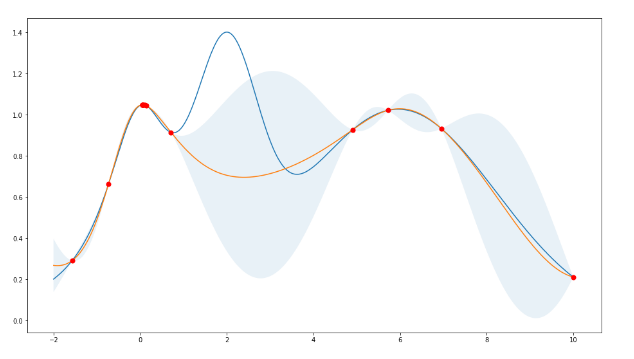图：贝叶斯超参数优化的高斯过程

5、结果

（1）绘制第一次训练之后的结果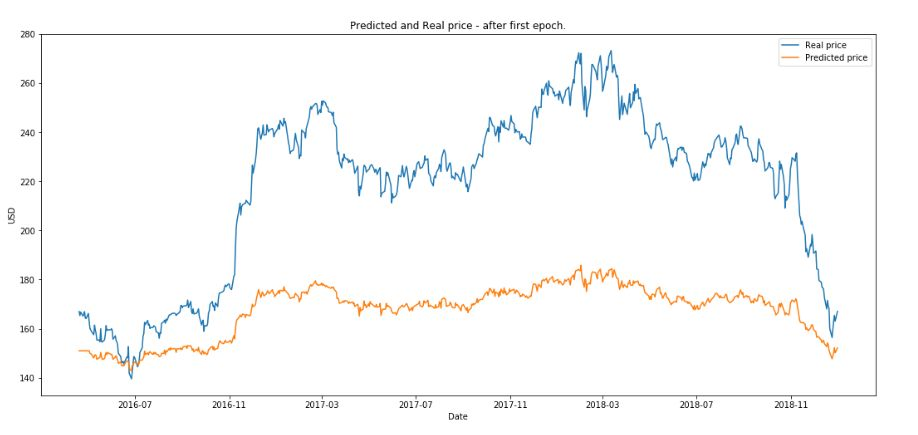（2）绘制 50 次训练后的结果。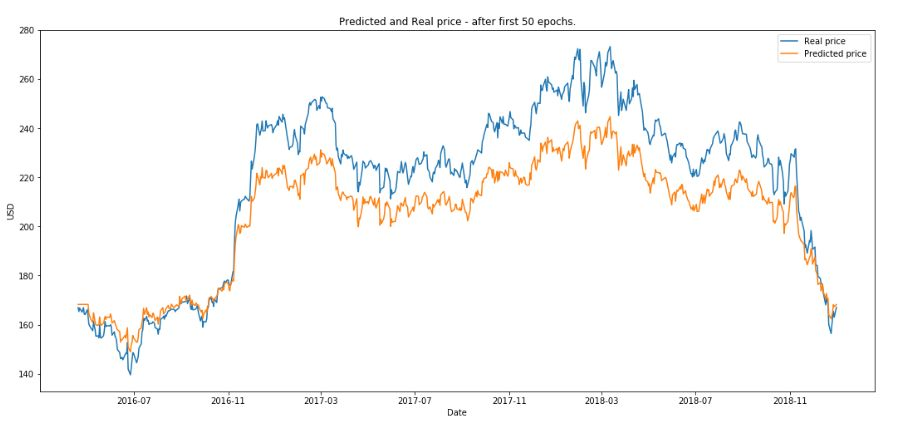（3）绘制 200 次训练后的结果。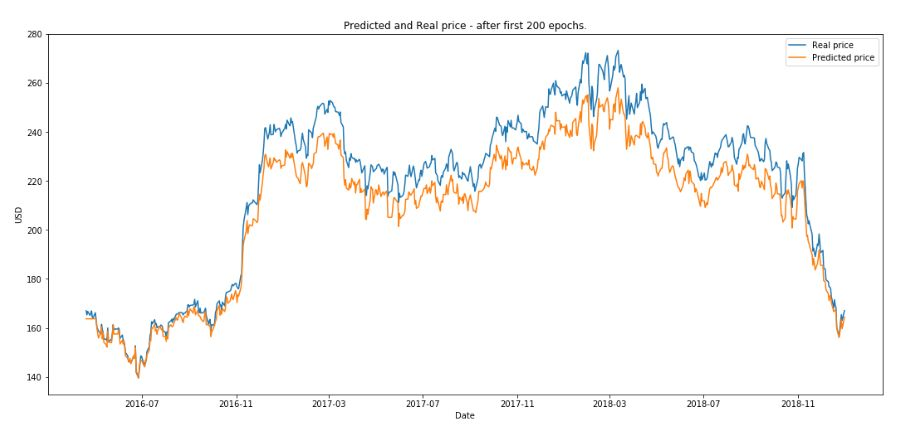RL 运行了 10 eposide ，本文定义一个 eposide 是 GAN 完整训练 200 次后，下图是得到的最终的结果。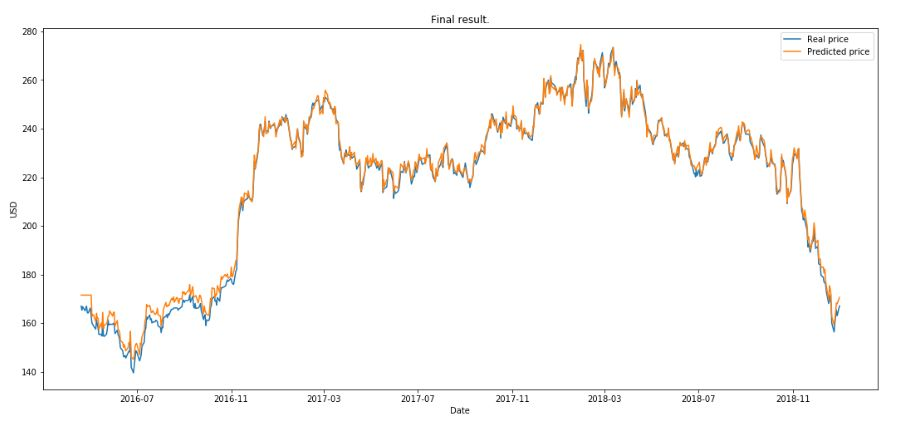https://github.com/borisbanushev/stockpredictionai

（本文为AI科技大本营编译文章，转载请微信联系1092722531

5月25-27日，由中国IT社区CSDN与数字经济人才发展中心联合主办的第一届CTA核心技术及应用峰会将在杭州国际博览中心隆重召开。首届CTA核心技术及应用峰会将围绕人工智能，邀请技术领航者与开发者共同探讨机器学习知识图谱的前沿研究及应用。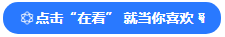08-13
09-275568
10-112677
06-015万+
04-275001
11-27323
01-144243
06-092456
06-222571
06-102983
06-073248
09-139837
11-081854
02-068145
01-02459
06-231352
07-291万+点击重新获取扫码支付余额充值